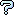All about flooble | fun stuff | Get a free chatterbox | Free JavaScript | Avatarsperplexus dot infoSquare of an Odd (Posted on 2002-10-06)Take any odd number and square it. It will invariably be a multiple of 8 plus 1. So (odd)^2=8n+1 where n is an integer. Show why this is always so. Also show what the pattern for n is.

 See The Solution Submitted by martyn Rating: 3.1333 (15 votes)Comments: ( Back to comment list | You must be logged in to post comments.)Similar problem| Comment 14 of 20 |I used to be fascinated that if you took an odd number, squared it, divided the square by two and added/subtracted a half from the result you achieve three integers which form the sides of a right angled triangle. Until I put it onto a spreadsheet and tried to iterate the problem. It falls apart at 27661, then again at 28339, 28589. Is this just the limitation of my spreadsheet package? Is there a sequence to these numbers? Why is the answer (x^2 + y^2 - z^2) always 32? Is this the meaning of life? Is it OK to ask this here, I'm new to this?
 Posted by Jonathan Fletcher on 2004-10-07 07:32:51Please log in:

 Search: Search body:
Forums (0)